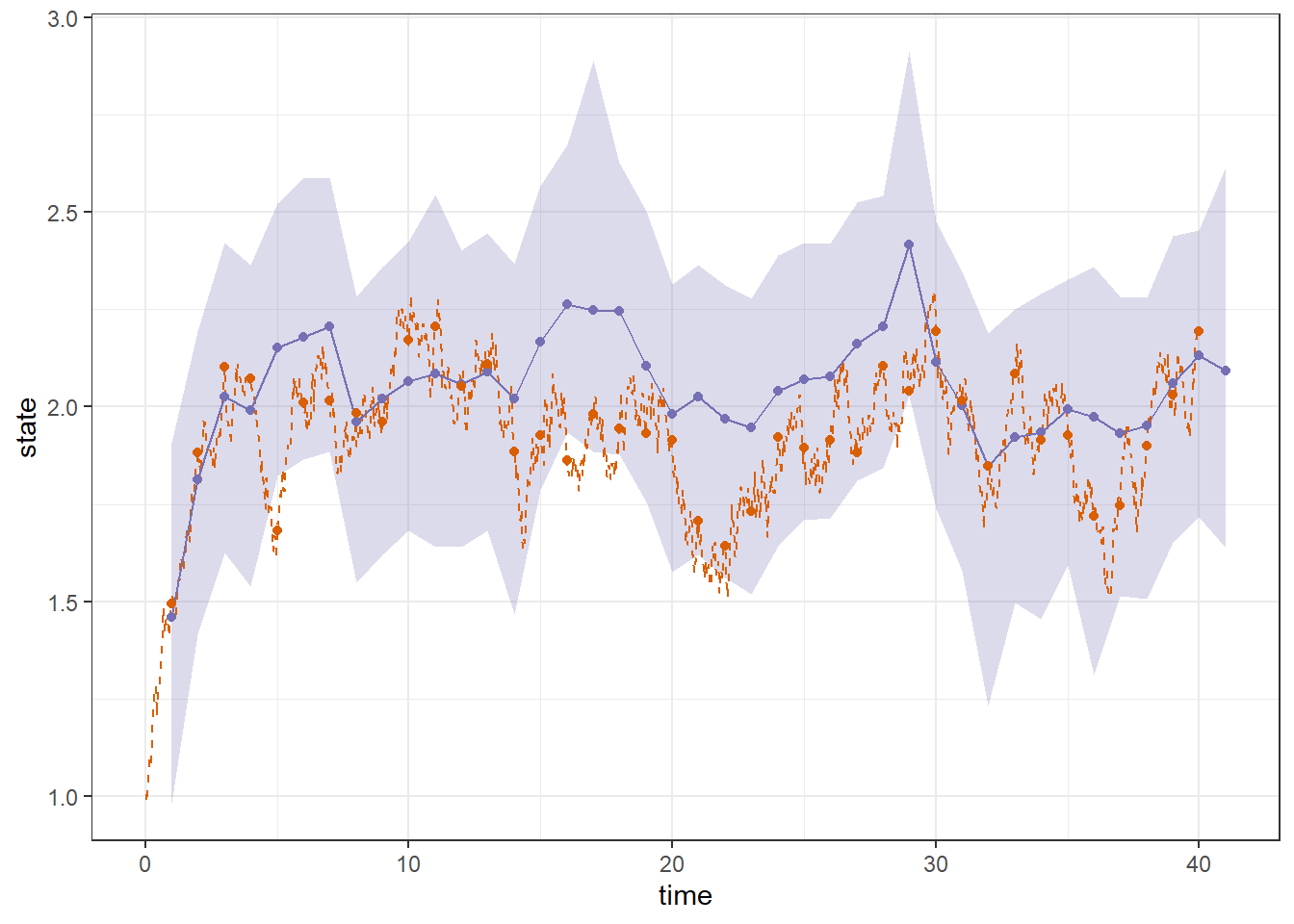## Introduction

This vignette shows how to model discretely observed diffusion models with bssm. We assume that the state equation is defined as a continuous time diffusion model of form $\textrm{d} \alpha_t = \mu(\alpha_t,\theta) \textrm{d} t + \sigma(\alpha_t, \theta) \textrm{d} B_t, \quad t\geq0,$ where $$B_t$$ is a Brownian motion and where $$\mu$$ and $$\sigma$$ are scalar-valued functions, with the univariate observation density $$g(y_k | \alpha_k)$$ defined at integer times $$k=1\ldots,n$$. As these transition densities are generally unavailable for non-linear diffusions, we use Milstein time-discretisation scheme for approximate simulation with bootstrap particle filter. Fine discretisation mesh gives less bias than the coarser one, with increased computational complexity. Here IS-MCMC approach can provide substantial computational savings.

## Example

Discretely observed latent diffusion models can be constructed using the ssm_sde function, which takes pointers to C++ functions defining the drift, diffusion, the derivative of the diffusion function, the log-densities of the observations, and the log-prior. As an example, let us consider an Ornstein–Uhlenbeck process $\textrm{d} \alpha_t = \rho (\nu - \alpha_t) \textrm{d} t + \sigma \textrm{d} B_t,$ with parameters $$\theta = (\log\rho, \nu, \log\sigma) = (\log(0.5), 2, \log(0.2))$$ and the initial condition $$\alpha_0 = 1$$. For observation density, we use Poisson distribution with parameter $$\exp(\alpha_k)$$. We first simulate a trajectory $$x_0, \ldots, x_{40}$$ using the sde.sim function from the sde package and use that for the simulation of observations $$y$$:

set.seed(1)
n <- 40
suppressMessages(library("sde"))
x <- sde.sim(t0 = 0, T = n, X0 = 1, N = n * 2^5,
drift = expression(0.5 * (2 - x)),
sigma = expression(0.2),
sigma.x = expression(0),
method = "milstein")
integer_x <- x[seq(frequency(x) + 1, length(x), frequency(x))]
y <- rpois(n, exp(integer_x))

We then modify the C++ functions (see Appendix) which define the terms of the stochastic differential equation, the observation density, and the priors for the unknown parameter vector $$\theta$$. After compilation with the help of Rcpp::sourceCpp, we input pointers to these functions to ssm_sde function:

library("bssm")
Rcpp::sourceCpp("ssm_sde_template.cpp")
## Warning in normalizePath(path.expand(path), winslash, mustWork):
## path="C:/Users/jvhels/AppData/Local/Temp/RtmpOcbFRh/Rbuild40c068197473/bssm/vignettes/../inst/include":
## The system cannot find the file specified
pntrs <- create_xptrs()
sde_model <- ssm_sde(y, pntrs$drift, pntrs$diffusion,
pntrs$ddiffusion, pntrs$obs_density, pntrs\$prior,
theta = c(log_rho = log(0.5), mu = 2, log_sigma = log(0.2)),
x0 = 1, positive = FALSE)

We then run IS-MCMC with 20,000 iterations (with first half discarded as burn-in by default), using coarse mesh with $$L_c=2^2$$ discretization points, finer mesh with $$L_f=2^5$$ points, and 30 particles. We also use two parallel threads for faster post-processing step with finer mesh (note that for accurate inference, more iterations should be used, but here we keep the run short and use only two threads due to CRAN check requirements).

out <- run_mcmc(sde_model, iter = 2e4, particles = 30, L_c = 2, L_f = 5, threads = 2)

Finally, we can draw our estimated state trajectory and the the corresponding 95 % posterior intervals, together with true process (dashed line, with points corresponding to integer times):

suppressMessages(library("ggplot2"))
suppressMessages(library("dplyr"))
suppressMessages(library("diagis"))

d <- as.data.frame(out, variable = "states")

state_fit <- d |>
group_by(time) |>
summarise(state = weighted_mean(value, weight),
lwr = weighted_quantile(value, weight, 0.025),
upr = weighted_quantile(value, weight, 0.975))

ggplot(state_fit, aes(x = time, y = state)) +
geom_ribbon(aes(ymin = lwr, ymax = upr),
fill = "#7570b3", alpha = 0.25) +
geom_line(data = data.frame(
state = x,
time = time(x)),
colour = "#d95f02", linetype = "dashed") +
geom_line(colour = "#7570b3") +
geom_point(colour = "#7570b3") +
geom_point(data=data.frame(state=integer_x,time=1:n), colour = "#d95f02") +
theme_bw()## Appendix

This is the full ssm_sde_template.cpp file:

// A template for building a univariate discretely observed diffusion model
// Here we define a latent Ornstein–Uhlenbeck process with Poisson observations
// d\alpha_t = \rho (\nu - \alpha_t) dt + \sigma dB_t, t>=0
// y_k ~ Poisson(exp(\alpha_k)), k = 1,...,n

// [[Rcpp::interfaces(r, cpp)]]

// x: state
// theta: vector of parameters

// theta(0) = log_rho
// theta(1) = nu
// theta(2) = log_sigma

// Drift function
// [[Rcpp::export]]
double drift(const double x, const arma::vec& theta) {
return exp(theta(0)) * (theta(1) - x);
}
// diffusion function
// [[Rcpp::export]]
double diffusion(const double x, const arma::vec& theta) {
return exp(theta(2));
}
// Derivative of the diffusion function
// [[Rcpp::export]]
double ddiffusion(const double x, const arma::vec& theta) {
return 0.0;
}

// log-density of the prior
// [[Rcpp::export]]
double log_prior_pdf(const arma::vec& theta) {

// rho ~ gamma(2, 0.5) // shape-scale parameterization
// nu ~ N(0, 4)
// sigma ~ half-N(0,1) (theta(2) is log(sigma))
double log_pdf =
R::dgamma(exp(theta(0)), 2, 0.5, 1) +
R::dnorm(theta(1), 0, 4, 1) +
R::dnorm(exp(theta(2)), 0, 1, 1) +
theta(0) + theta(2); // jacobians of transformations
return log_pdf;
}

// log-density of observations
// given vector of sampled states alpha
// [[Rcpp::export]]
arma::vec log_obs_density(const double y,
const arma::vec& alpha, const arma::vec& theta) {

arma::vec log_pdf(alpha.n_elem);
for (unsigned int i = 0; i < alpha.n_elem; i++) {
log_pdf(i) = R::dpois(y, exp(alpha(i)), 1);
}
return log_pdf;
}

// Function which returns the pointers to above functions (no need to modify)

// [[Rcpp::export]]
Rcpp::List create_xptrs() {
// typedef for a pointer of drift/volatility function
typedef double (*fnPtr)(const double x, const arma::vec& theta);
// typedef for log_prior_pdf
typedef double (*prior_fnPtr)(const arma::vec& theta);
// typedef for log_obs_density
typedef arma::vec (*obs_fnPtr)(const double y,
const arma::vec& alpha, const arma::vec& theta);

return Rcpp::List::create(
Rcpp::Named("drift") = Rcpp::XPtr<fnPtr>(new fnPtr(&drift)),
Rcpp::Named("diffusion") = Rcpp::XPtr<fnPtr>(new fnPtr(&diffusion)),
Rcpp::Named("ddiffusion") = Rcpp::XPtr<fnPtr>(new fnPtr(&ddiffusion)),
Rcpp::Named("prior") = Rcpp::XPtr<prior_fnPtr>(new prior_fnPtr(&log_prior_pdf)),
Rcpp::Named("obs_density") = Rcpp::XPtr<obs_fnPtr>(new obs_fnPtr(&log_obs_density)));
}
Iacus, Stefano Maria. 2016. Sde: Simulation and Inference for Stochastic Differential Equations. https://CRAN.R-project.org/package=sde.
Vihola, Matti, Jouni Helske, and Jordan Franks. 2020. “Importance Sampling Type Estimators Based on Approximate Marginal MCMC.” Scandinavian Journal of Statistics. https://doi.org/10.1111/sjos.12492.